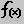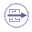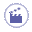# Creating Points, Lines... as ParametersThe scenario below explains how to determine the position of the inertia axis of a pad. To do so, start from a pad, then:
1. Create a line by using either method ('datum' or)
2. Use the inertiaAxis line constructor to specify that this line is to be the inertia axis of the pad.
3. Retrieve the coordinate of the point located at the intersection of the inertia axis and the pad extrusion plane.To create elements such as  Points, Lines, Curves, Surfaces, Planes or Circles and use them in knowledgeware relations, you can:
 Create these elements as 'Isolate' elements in the Generative Shape Design workbench. 'Isolate' elements also called Datum are elements that have no link to the other entities that were used to create them.  For information on 'Datum' type elements, see the Generative Shape Design User's Guide. Create these elements by using the f(x) capabilities and select the right type of element from the New parameter of type list.1. Access the Part Design workbench, create any sketch in the yz plane, then extrude this sketch to create a pad. If need be, refer to the Part Design User's Guide.

2. Create a line intended to be used as an inertia axis afterwards.

3. To do so, click the Formulas icon , select the Line item in New Parameter of type, then click New Parameter of type.

4. Click the Formulas icon. From the parameter list, select the line you have created (Geometrical Set.1\Line.1).

5. Click Add Formula and add the formula below in the editor:
```Geometrical Set.1\Line.1 = inertiaAxis(3,PartBody) ```The inertiaAxis function is accessible through the Line constructors. The axis number 3 is the one which is in the extrusion direction (normal to yz). Click OK in the Formulas dialog box. The inertia axis is displayed in the geometry area.

6. Back to. Create three length type parameters: X, Y and Z.

7. Retrieve the coordinates of the point located at the intersection of the inertia axis and the 'yz plane'. To do so, create the formulas below:

 ```X=intersect(Geometrical Set.1\Line.1, 'yz plane').coord(1) Y=intersect(Geometrical Set.1\Line.1, 'yz plane').coord(2) Z=intersect(Geometrical Set.1\Line.1, 'yz plane').coord(3)```

You get the intersect  function from the Wireframe constructors and the point.coord method from the Measures item of the dictionary.

8. Check the value displayed in the specification tree as well as in the Formulas dialog box.

The KwoGettingStarted.CATPart document used as a sample for the Product Engineering Optimizer User's Guide illustrates this scenario.